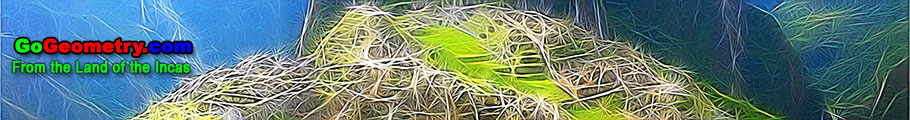###### Online Geometry Problem 870: Triangle, Median, Three Squares, Centers, Vertices, Collinear Points, Midpoint. Level: High School, College, Mathematics Education
 The figure below shows a triangle ABC with the median BN. If ABDE, BFGN, and BHMC are squares of centers O1, O2, and O3, respectively, prove that (1) F is the midpoint of DH, (2) G is the midpoint of EM, (3) O2, is the midpoint of O1O3.Home | Search | Geometry | Problems | All Problems | Open Problems | Visual Index | 10 Problems | 861-870 | Square | Median | Collinear Points | Midpoint | Email | Solution / comment | By Antonio Gutierrez